Chapter 11.7, Problem 60E

Chapter
Section
Textbook Problem

# MatchingIn Exercises 71–76, match the equation (written in terms of cylindrical or spherical coordinates) with its graph. [The graphs are labeled (a), (b), (c), (d), (e), and (f).](a)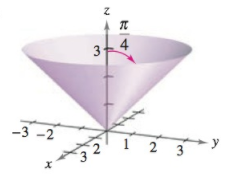(b)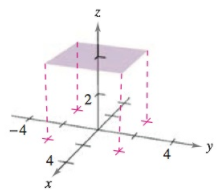(c)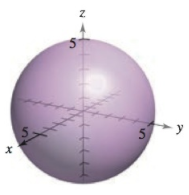(d)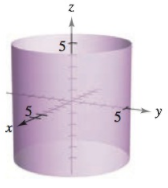(e)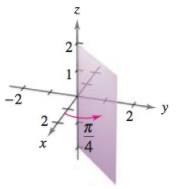(f)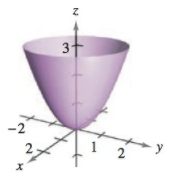ϕ = π 4

To determine
For the given graphs, find the appropriate graph among the given graphs for the equation φ=π4.

Explanation

Given:

The following provided graphs are:

Explanation:

Convert spherical equation to rectangular equation.

The given equation is a spherical equation,

Standard

φ=arccoszx2+y2+z2

As the equation is φ=π4.

Put values,

arccoszx2+y2+z2=π4zx2+y2+z2<

### Still sussing out bartleby?

Check out a sample textbook solution.

See a sample solution

#### The Solution to Your Study Problems

Bartleby provides explanations to thousands of textbook problems written by our experts, many with advanced degrees!

Get Started